# A More Exact Coax Attenuation Solution

New for December 2012: we've applied the more exact solution in looking at wirebond attenuation versus frequency.

First posted in September 2006, this page shows something that we've never seen in a textbook or web site or IEEE paper, a calculation of coax attenuation versus frequency that makes no assumptions about how many skin depths of metal you have in each conductor. A calculation that Agilent's ADS gets wrong! Caution: the material on this page might be useful under certain conditions, but it might also be considered nerdly by your spouse so try not to bring up the subject during dinner at that fancy restaurant with your in-laws who are in town glomming a free meal from your Mastercard... just keep tipping them back until someone cuts you off...

The"textbook" calculation for coax metal attenuation makes the assumption that the skin depth is much less than the inner conductor diameter (dimension"a" below), or the thickness of the outer conductor (dimension"c" minus dimension"b" below). So what happens if this is not the case? Suppose the skin depth is a significant fraction (or even greater than) the critical dimensions of the conductors? This can happen when you put a 1 MHz signal through a skinny coax line. At lower and lower frequencies, the skin depth gets greater and greater (it goes as 1/SQRT(f)), and at some point this assumption is no longer valid. Pay attention, owners of AM radio stations.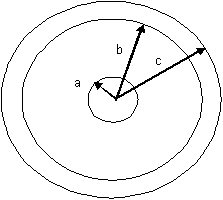Let's look at the textbook solution to coax attenuation. Before we calculate the resistance per unit length of the two conductors, we'll first just look at how the equivalent cross-sectional area of the two conductors is calculated (an intermediate step on the way to calculating resistance/length). Remember that RF sheet resistance is calculated from an area of conductor that has depth equal to one skin depth. For very small skin depth (according to microwave textbooks):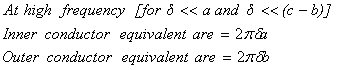What happens when you misapply this equation at lower frequencies? At DC (0 Hertz), the skin depth is infinity. The solution above can't be correct, or your coax would have infinite conductivity, and zero resistance.

### The more exact solution

Before we get started we must stress that errors under"normal" microwave frequencies are quite small when the above equation is applied. Now then...

The figure above defines the dimensions for this little math problem. The center conductor is radius a, the inside of the outer conductor is radius b, and the outside diameter of the outer conductor is radius c. The math associated with the exact solution is an integration problem, noting that as you penetrate into the conductor the current density goes down as EXP(-z/δ), where δ is the skin depth. We'll save the integration for an IEEE letter or something scholarly, and not show it here for now. Plus, it makes a great final exam problem for microwave students so we don't want to give the entire solution away!

After integrating and simplifying, the solutions for the equivalent area of the center conductor and the outer conductor are: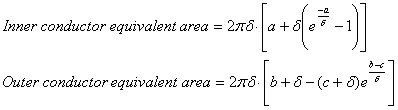Note that skin depths on inner and outer conductor should always be considered separately, as they are not always of the same material.

How do we know this solution is valid? There's two sniff tests. At DC, the area should equal the geometric area of the center conductor and outer conductor"donut", which is an eighth grade geometry problem: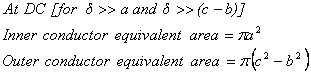And second, at high frequency the textbook solution should be satisfied. Looking at the equations, it is not at all obvious that we have succeeded, so it is time to make some plots.

From here the solution to determining metal loss is easy, first you convert the equivalent areas of the conductors to resistance/length: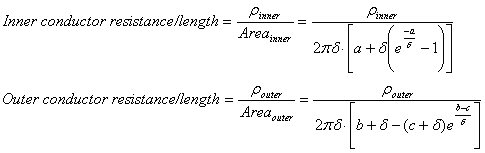Then the attenuation due to metal can be found by noting: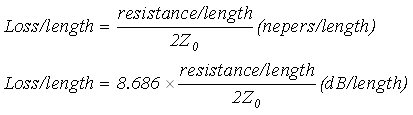We're not going to show a closed form equation that does this all in one step (at least for now). This type of math is best done using Excel, then you don't have to solve the entire problem in one step. If you go to our download area and grab coax.xls we've entered these equations and tons more into a versatile spreadsheet for studying coax.

### Example: a=1mm, b=2.3mm, c=2.4mm

This is a fifty ohm line if air is used as the dielectric. First let's look at the equivalent area of the center conductor. We've entered our more exact equations into a spreadsheet that also calculates skin depth over frequency, and plotted the equivalent area over many decades of frequency using a log scale. The blue line is the textbook calculation, below 1 MHz it completely overestimates the equivalent area. Note that the new equation converges to the correct DC value which is shown in red (the"geometric area").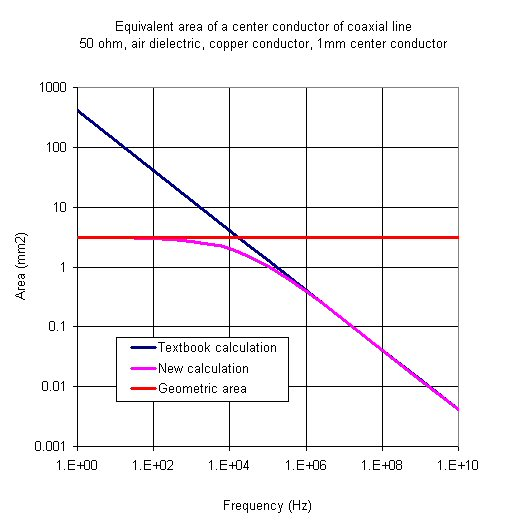Here's the outside conductor area, it also meets the sniff tests of getting the DC area correct and converging to the classic textbook solution at microwave frequencies: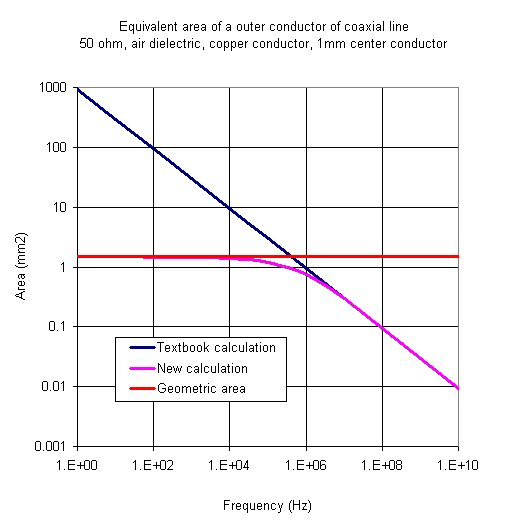Here let's look at the total equivalent area of both center and outer conductors.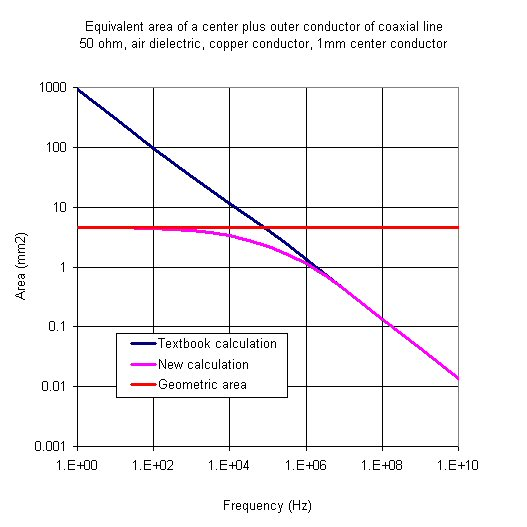Now let's look at the resistance/length. This is simply the bulk resistivities of the conductors divided by their cross-sectional areas which we just calculated. In the calculation we assumed both conductors are copper. Be sure to keep your units straight, in the area calculations we used millimeters2, so the area have to be divided by 1,000,000 to get to meters2.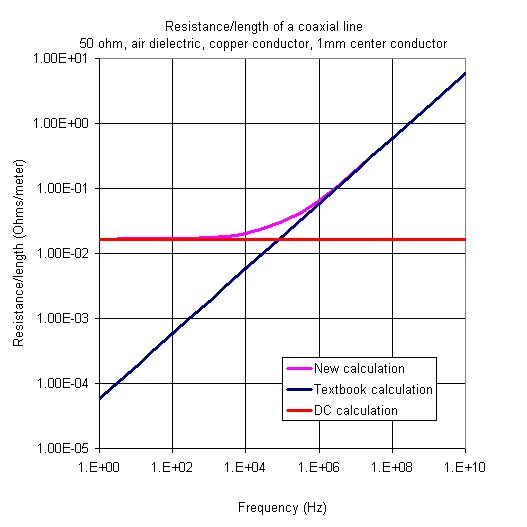The bottom line is that the textbook calculation will always give zero ohms/length at DC, while the new calculation gives the correct value which is a very small number (less than 0.02 ohms per meter total for both conductors). The error is so small it can be ignored, unless you are running miles of cable with signals below 1 MHz!

BTW, how do we know that Agilent's ADS doesn't get this calculation right? Look at their circuit model below. It doesn't specify a thickness for the outer conductor! This means that the outer conductor is assumed infinite thickness. We have heard that the center conductor is modeled with the calculation that we show here, so at best the boyz at Agilent have their model half right! But again we must stress that the error under"normal" microwave frequencies is quite small.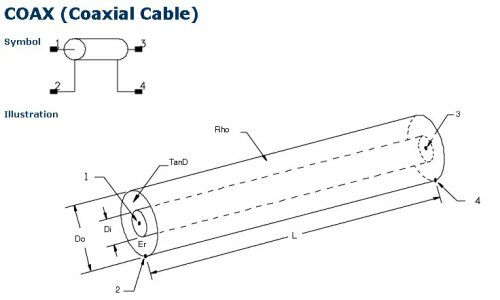Agilent's coax model

Go to our download page and get the coax spreadsheet that does this calculation and so much more!

More to come!

Author : Unknown Editor